Home Practice
For learners and parents For teachers and schools
Textbooks
Mathematics
JSS 1 JSS 2 JSS 3
Full catalogue
Pricing SupportLog in

We think you are located in Nigeria. Is this correct?

# Test yourself now

High marks in science are the key to your success and future plans. Test yourself and learn more on Siyavula Practice.

# Chapter 11: Simple equations

You have learnt that an algebraic expression is a mathematical phrase or statement. Algebraic expressions do not have equals signs. In this chapter we will be dealing with algebraic equations. Algebraic equations are mathematical sentences with equals signs. You will learn how to formulate and solve algebraic equations.

## 11.1 Formulating equations

An algebraic equation is a mathematical sentence that contains a combination of numbers, symbols, variables and mathematical operators. It always has an equals sign.

algebraic equation An algebraic equation is a mathematical sentence that contains a combination of numbers, symbols, variables and mathematical operators. It always has an equals sign.

### The difference between expressions and equations

It is important that you understand the difference between algebraic expressions and algebraic equations. The table below lists the main differences.

Expression Equation
An expression is a phrase. An equation is a sentence.
We simplify an expression. We solve an equation.
An expression does not have an equals sign. An equation has an equals sign.
An expression gives the relationship between variables. An equation shows that two expressions are equal.
The variables in an expression can have a whole range of values. The variables in an equation only has specific values.
Example: Two more than a number, written as $x+2$ Example: Two more than a number is five, written as $x+2=5$

Let's look at an example from Chapter 10 to explain this further.

Suppose a shop owner sells maize at $$₦\,x$$ per cup. She also sells rice per cup, at double the price of the maize. On a specific day, she sold 50 cups of maize and 45 cups of rice.

• We can write an expression for her income for the day: $50\times x+45\times 2x$
• We can simplify the expression to $50x+90x$, and simplify further to: $140x$
• Her income for the day is therefore: $$₦\,140x$$
• Her income depends on the cost of a cup of maize, which can be any positive number.
• If we know that her income for the day was $$₦\,7,700$$, we can write an equation: $50\times x+45\times 2x = 7,700$
• We can simplify the equation to: $140x=7,700$
• The only value that makes this sentence true is $55$.
• Now we know that the price of a cup of maize is $$₦\,55$$ and the price of a cup of rice is $$2\times55=₦\,110$$.

### Translate word sentences into algebraic equations

In Section 9.3 of Chapter 9 and Section 10.6 of Chapter 10 you were given some examples of ways to write word phrases as mathematical statements. Revise those sections before you go on.

### Worked example 11.1: Translating simple word sentences into algebraic equations

If a number is multiplied by 4, the answer is 6 more than the number. Write an equation to represent this.

1. Step 1: Identify the unknown and make it $x$.

In this case, the unknown is the number. So, we say the number is $x$.

2. Step 2: Write an expression or value for one part of the equation.

One of the phrases states that the number is multiplied by 4. We write this as $4x$.

3. Step 3: Write an expression or value for the other part of the equation.

Another phrase refers to 6 more than the number. We write this as $x+6$.

4. Step 4: Remember than an equation has an equals sign. Decide how the two expressions are equal to each other.

The words say the answer of the multiplication by 4 gives the addition of 6. Therefore:

If we solve the equation above, we get $x=2$. You will learn how to solve equations in the next section.

### Worked example 11.2: Translating more complex word sentences into algebraic equations

A mother is twice as old as her daughter. In two years' time, their combined ages will be 52 years. Write an equation to represent this.

1. Step 1: Identify the unknowns.

There are two unknowns:

• The mother's age
• The daughter's age
2. Step 2: Make the one unknown $x$. Write an expression for the other unknown in terms of $x$.

• Make the daughter's age $x$.
• The mother's age is twice that. Therefore, the mother's age is $2x$.

We could also say the mother's age is $x$ and the daughter's age is $\frac{1}{2}x$. However, it is often easier to solve equations with whole numbers as coefficients than equations with fractions as coefficients.

3. Step 3: Write an expression or value for one part of the equation.

One phrase refers to their ages in two years' time:

• The daughter will be $x+2$ years old.
• The mother will be $2x+2$ years old.
4. Step 4: Write an expression or value for the other part of the equation.

Another phrase says their combines ages will be 52.

5. Step 5: Remember than an equation has an equals sign. Decide how the two expressions are equal to each other.

Their combined ages in two years' time is equal to 52. If we combine their ages, this means we add the ages. Therefore:

If we solve the equation above, we get $x=16$. This means the daughter is 16 years old and the mother is 32 years old. You will learn how to solve equations in the next section.

### Exercise 11.1: Translate word sentences into equations

Write an algebraic equation for each of the following situations.

1. If we add 23 to a number, the answer is 42.

2. The difference between 75 and a number is 20.

3. A pen costs twice as much as a ruler. The cost of 5 pens and 7 rulers is $$₦\,102$$.

4. If we add 9 to half of a number, the answer is twice the number.

5. The sum of three consecutive numbers is 24.

6. The length of a rectangle with a perimeter of 40 cm is three times more than its breadth.

7. The combined area of two rectangles is 49 square units. The area of one rectangle is six times more than the area of the other rectangle.

8. If the sum of a number and 6 is divided by 10, the answer is 24.

9. Danjuma has $$₦\,500$$. He spends a certain amount on snacks and exactly the same amount on data. He then spends twice the amount he spent on snacks to buy stationery. He has $$₦\,40$$ left.

10. Ngozi goes to school. She walks for a few minutes and then runs for 180 seconds. Then she walks again for half the time she walked in the beginning. Her journey takes 15 minutes.

If we work in seconds:

If we work in minutes:

### Translate algebraic equations into word sentences

We can also translate algebraic equations into word sentences. You can make up a story if you want to, or you can just think of the variable as a number.

### Worked example 11.3: Translating algebraic equations into word sentences

Translate the following equation into a word sentence:

1. Step 1: Assume that the variable is a number.

In this case the variable is $y$. So we assume that $y$ is a number.

2. Step 2: Interpret one part of the equation.

$3+y$ means we add a number to 3.

3. Step 3: Interpret the other part of the equation.

$10-2y$ means we subtract twice the number from 10. We can also say it is the difference between 10 and twice the number.

4. Step 4: Say how the two parts are equal to each other.

When we add 3 to a number, the answer is equal to the difference between 10 and twice the number.

### Exercise 11.2: Translate equations into word sentences

Write a word sentence for each of the following algebraic equations.

1. If we add 12 to a number, the answer is 25.

2. The difference between twice a number and 8 is 30.

3. Two thirds of a number is equal to 12.

4. If we subtract a number and three times the same number from 120, the answer is 60.

5. The sum of twice a number and half of the same number is 10.

6. The difference between 8 times a number and a quarter of the same number is 62.

7. The sum of twice a number and five times the same number is equal to 10 less than the number.

8. When the difference between a number and 10 is divided by 4, the answer is 20.

9. The sum of twice a number and four times the same number is 30.
OR
The length of a rectangle with a perimeter of 30 units is twice its breadth.

## 11.2 Solving equations

### The solution of an equation

You have learnt how to find the values that make open sentences true. Look at the following algebraic equation:

It is easy to see that the only value for $x$ that will make this equation true is 2. This is because $3+2=5$. We say the number 2 is the solution of the equation and we write it as:

When the find the solution of an equation, we say we solve the equation.

solution The solution of an equation is the value of the variable that makes the equation true.

solve When we solve an equation, we find the value of the variable that makes the equation true.

To solve an equation, we must isolate the variable. If $x$ is the variable, we must get the equation in the form:

### Representing an equation as a balancing scale

To help us solve an algebraic equation, we can show it as a balancing scale. The picture shows what a scale looks like. This scale is balanced, because nothing has been placed on it.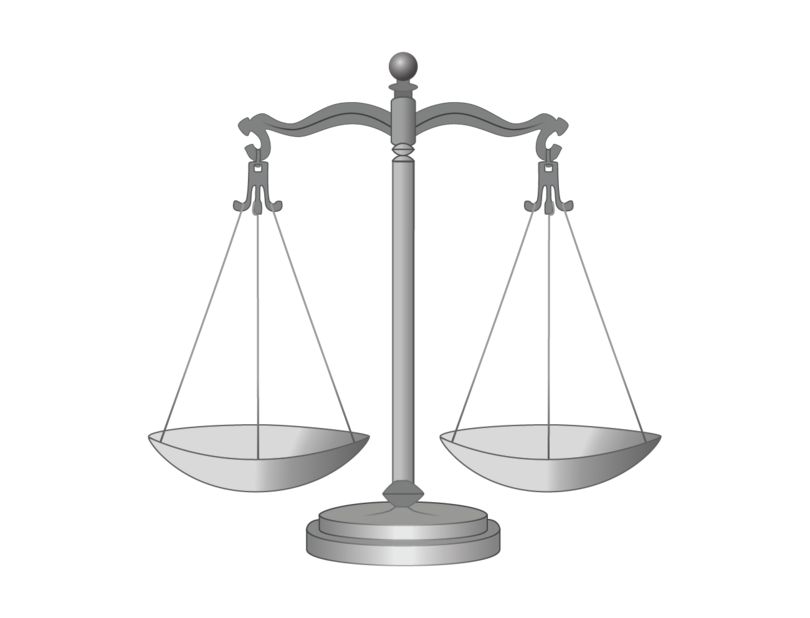If we place a mass piece on one of the pans, the scale becomes unbalanced.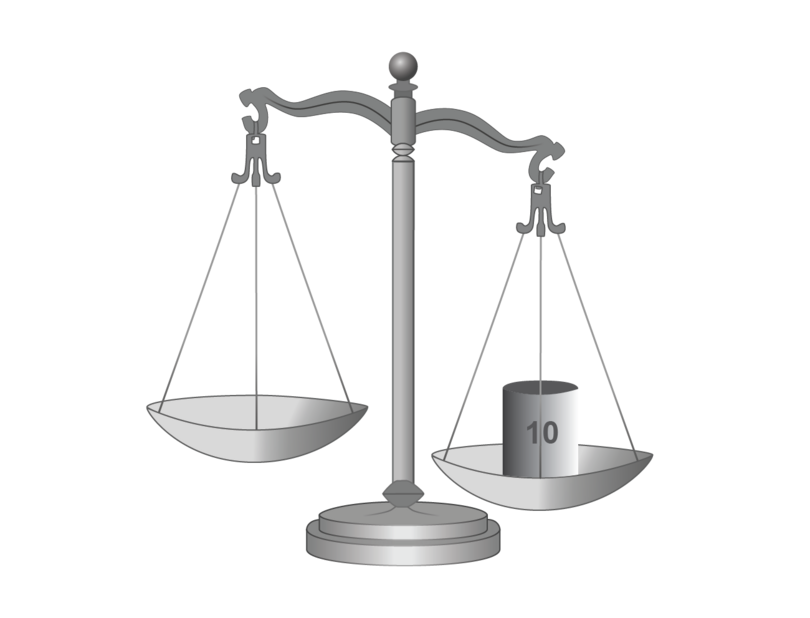We can balance the scale again by placing an identical mass piece on the other pan.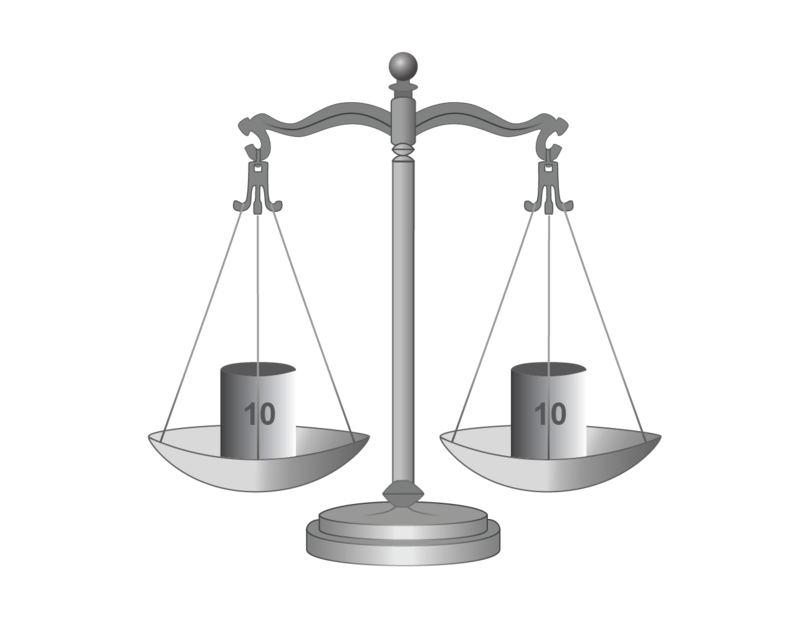As long as identical mass pieces are placed on both pans, the scale will remain in balance.

In an equation, the equals sign shows that two expressions (or an expression and a value) are equal to each other. We can think of each side of the equation as one of the pans of a scale. As long as we do exactly the same on each side of the equation, the equation will remain true.

Consider the following algebraic equation:

We can represent it on the pans of a scale as follows: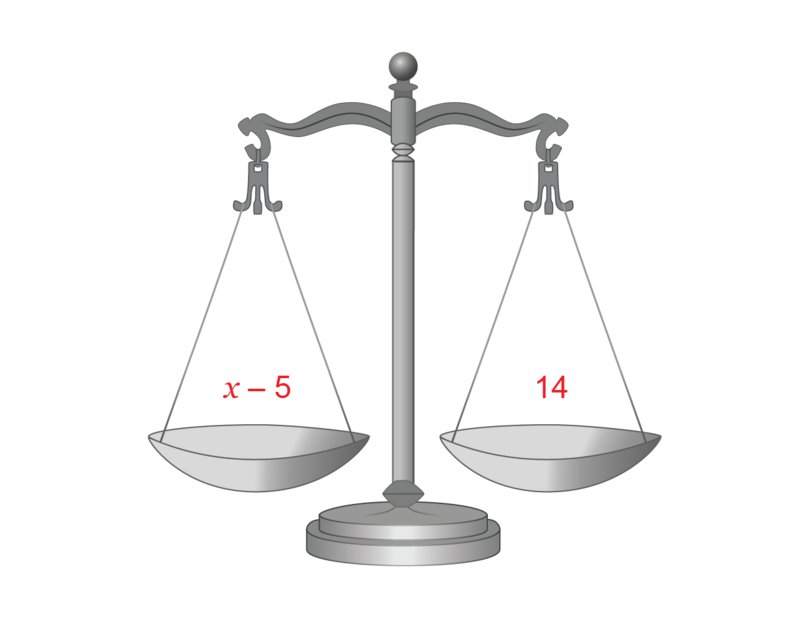Our aim is to isolate the variable. We must therefore "take away" the -5 from the left-hand scale. To do this, we add the additive inverse of -5.

Go back to Chapter 9 if you need to revise inverse operations. Remember that addition and subtraction are inverse operations, and multiplication and division are inverse operations.

Whatever we add to the one pan, we must also add to the other pan. The scale now shows: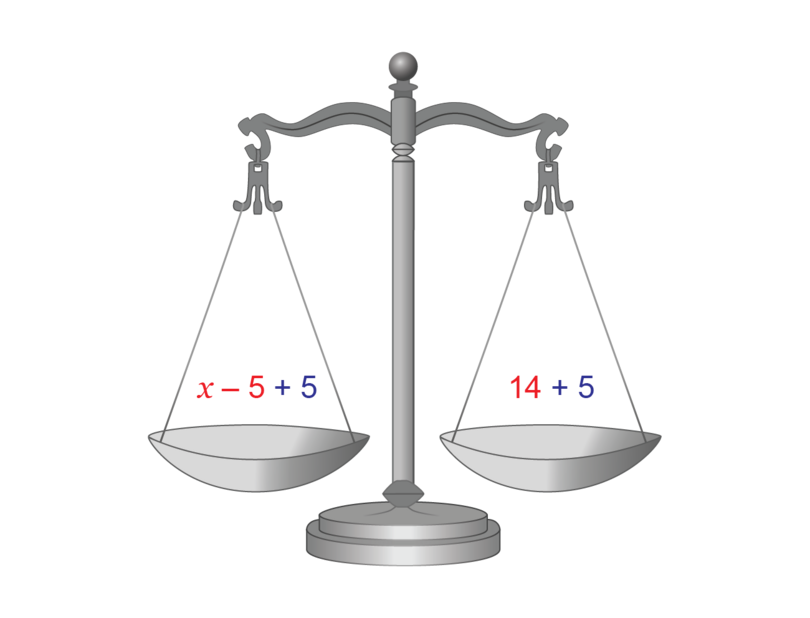Remember that $-5+5=0$. Therefore, if we simplify both sides, we get the solution of the equation:

### Worked example 11.4: Finding the solution of an equation

Solve the following equation:

1. Step 1: If either side of the equation can be simplified, do that first. On the left side, you can add like terms.

\begin{align} 2x+x-10&=2 \\ 3x-10&=2 \end{align}
2. Step 2: If there is a constant term on the same side as the variable, add its additive inverse. Remember to do this on both sides of the equation.

\begin{align} 2x+x-10&=2 \\ 3x-10\; \color{red}{+\;10}&=2\; \color{red}{+\;10} \\ 3x&=12 \end{align}
3. Step 3: If the variable has a coefficient, apply the multiplicative inverse of the coefficient. Remember to do this on both sides of the equation.

Remember, multiplication and division are inverse operations. For example, $\frac{4}{1}\times \frac{1}{4} = \frac{4}{4}=1$

\begin{align} 2x+x-10&=2 \\ 3x-10\; \color{red}{+\;10}&=2\; \color{red}{+\;10} \\ 3x&=12 \\ \frac{3x}{\color{red}{3}}&=\frac{12}{\color{red}{3}} \\ \therefore x&=4 \end{align}

### Checking the solution

We always check the solution we worked out to make sure that it makes the equation true. An equation is true if the left-hand side is equal to (has the same value as) the right-hand side.

### Worked example 11.5: Checking the solution

In the previous worked example, the solution of the equation:

was found to be:

Check this solution.

1. Step 1: Replace the variable on the left-hand side (LHS) of the original equation with the solution. Work out the value of the left-hand side.

\begin{align} \text{LHS}&=2x+x-10 \\ &=2(4)+4-10 \\ &=8+4-10 \\ &=2 \end{align}

Remember that $2(4)$ means $2\times 4$.

2. Step 2: Repeat Step 1 for the right-hand side (RHS) of the original equation.

3. Step 3: Compare your answers from Steps 1 and 2. If they are the same, your solution is correct. If not, you made a mistake somewhere. Solve the equation again.

$\text{LHS}=\text{RHS}$
The solution is correct.

### Exercise 11.3: Solve equations and check the solutions

Solve each of the following equations and check the solutions.

1. \begin{align} \frac{11x}{11}&=\frac{33}{11} \newline \therefore x&=3 \end{align}

Checking:

\begin{align} 11(3)&=33 \newline 33&=33 \newline \therefore \text{LHS}&=\text{RHS} \end{align}
2. \begin{align} 18-18+p&=6-18 \newline \therefore p&=-12 \newline \end{align}

Checking:

\begin{align} 18+(-12)&=6 \newline 6&=6 \newline \therefore \text{LHS}&=\text{RHS} \end{align}
3. \begin{align} \frac{4}{1}\times \frac{1}{4}y&=\frac{4}{1}\times5 \newline y&=\frac{4\times5}{1} \newline \therefore y&=20 \end{align}

Checking:

\begin{align} \frac{1}{4}(20)&=5 \newline \frac{1\times 20}{4}&=5 \newline 5&=5 \newline \therefore \text{LHS}&=\text{RHS} \end{align}
4. \begin{align} 4x+5-5&=17-5 \newline 4x&=12 \newline \frac{4x}{4}&=\frac{12}{4} \newline \therefore x&=3 \end{align}

Checking:

\begin{align} 4(3)+5&=17 \newline 12+5&=17 \newline 17&=17 \newline \therefore \text{LHS}&=\text{RHS} \end{align}
5. \begin{align} 10-10+5a&=40-10 \newline 5a&=30 \newline \frac{5a}{5}&=\frac{30}{5} \newline \therefore a&=6 \end{align}

Checking:

\begin{align} 10+5(6)&=40 \newline 10+30&=40 \newline 40&=40 \newline \therefore \text{LHS}&=\text{RHS} \end{align}
6. \begin{align} 13+3&=2x-3+3 \newline 16&=2x \newline \frac{16}{2}&=\frac{2x}{2} \newline \therefore 8&=x \newline \therefore x&=8 \end{align}

$8=x$ and $x=8$ mean exactly the same thing.

Checking:

\begin{align} 13&=2(8)-3 \newline 13&=16-3 \newline 13&=13 \newline \therefore \text{LHS}&=\text{RHS} \end{align}
7. \begin{align} \frac{1}{2}t+1-1&=5-1 \newline \frac{1}{2}t&=4 \newline \frac{2}{1}\times \frac{1}{2}t&=4\times \frac{2}{1} \newline \therefore t&=8 \end{align}

Checking:

\begin{align} \frac{1}{2}t+1&=5 \newline \frac{1}{2}(8)+1&=5 \newline \frac{1 \times 8}{2}+1&=5 \newline 4+1&=5 \newline 5&=5 \newline \therefore \text{LHS}&=\text{RHS} \end{align}
8. \begin{align} 36-36+\frac{3}{4}y&=44-36 \newline \frac{3}{4}y&=8 \newline \frac{4}{3}\times \frac{3}{4}y&=8\times \frac{4}{3} \newline y&=\frac{8\times4}{3} \newline \therefore y&=\frac{32}{3} \newline \end{align}

Checking:

\begin{align} 36+\frac{3}{4} \left(\frac{32}{3}\right) &=44 \newline 36+\frac{3\times 32}{4 \times3}&=44 \newline 36+\frac{3\times 32}{3 \times4}&=44 \newline 36+\frac{1\times 8}{1 \times1}&=44 \newline 36+8&=44 \newline 44&=44 \newline \therefore \text{LHS}&=\text{RHS} \end{align}

Go back to Chapter 6 to revise how to multiply and divide fractions.

9. \begin{align} 1+9x&=19 \newline 1-1+9x&=19-1 \newline 9x&=18 \newline \frac{9x}{9}&=\frac{18}{9} \newline \therefore x&=2 \newline \end{align}

Checking:

\begin{align} 1-2(2)+11(2)&=19 \newline 1-4+22&=19 \newline 23-4&=19 \newline 19&=19 \newline \therefore \text{LHS}&=\text{RHS} \end{align}

Remember that you must always follow the correct order of operations: BODMAS (Brackets, Of, Division, Multiplication, Addition, Subtraction)

10. \begin{align} 39&=21+7q-4q \newline 39&=21+3q \newline 39-21&=21-21+3q \newline 18&=3q \newline \frac{18}{3}&=\frac{3q}{3} \newline 6&=q \newline \therefore q&=6 \end{align}

Checking:

\begin{align} 39&=21+5(6)-4(6)+2(6) \newline 39&=21+30-24+12 \newline 39&=63-24 \newline 39&=39 \newline \therefore \text{LHS}&=\text{RHS} \end{align}

### Equations with variables on both sides of the equals sign

Consider the following equation:

To solve an equation, we must isolate the variable. We must get the equation in the form:

In all the equations we have solved so far, the terms with variables were on one side of the equation only. The equation above has a term with a variable on each side of the equation. We need to work with the terms until there is a variable only on the left-hand side or the right-hand side of the equation. There are two ways to do this, as shown in the following worked example.

### Worked example 11.6: Solving an equation with variables on both sides

Solve the following equation:

1. Option 1: Use the additive inverse of a term with a variable to add or subtract it from both sides. We will start on the left-hand side.

\begin{align} 18-5x\; \color{red}{+\;5x}&=4x \; \color{red}{+\;5x} \newline 18 &= 9x \newline \frac{18}{9}&=\frac{9x}{9} \newline 2&=x \newline \therefore x&=2 \end{align}
2. Option 2: We will also use the additive inverse, but starting on the right-hand side.

\begin{align} 18-5x\; \color{red}{-\;4x}&=4x \; \color{red}{-\;4x} \newline 18-9x &= 0 \newline 18-9x\; \color{green}{+\;9x}&=0+\; \color{green}{9x} \newline 18&=9x \newline \frac{18}{9}&=\frac{9x}{9} \newline 2&=x \newline \therefore x&=2 \end{align}

### Exercise 11.4: Solve equations with variables on both sides

Solve each of the following equations and check the solutions.

1. \begin{align} 2x-2x+9&=5x-2x \newline 9&=3x \newline \frac{9}{3}&=\frac{3x}{3} \newline 3&=x \newline \therefore x&=3 \end{align}

Checking:

\begin{align} 2(3)+9&=5(3) \newline 6+9&=15 \newline 15&=15 \newline \therefore \text{LHS}&=\text{RHS} \end{align}
2. \begin{align} 8+4p-4p&=6p-4p-12 \newline 8&=2p-12 \newline 8+12&=2p-12+12 \newline 20&=2p \newline \frac{20}{2}&=\frac{2p}{2} \newline 10&=p \newline \therefore p&=10 \end{align}

Checking:

\begin{align} 8+4(10)&=6(10)-12 \newline 8+40&=60-12 \newline 48&=48 \newline \therefore \text{LHS}&=\text{RHS} \end{align}
3. \begin{align} 6a+7&=4a+13 \newline 6a-4a+7&=4a-4a+13 \newline 2a+7&=13 \newline 2a+7-7&=13-7 \newline 2a&=6 \newline \frac{2a}{2}&=\frac{6}{2} \newline \therefore a&=3 \end{align}

Checking:

\begin{align} 5(3)+7+3&=4(3)+13 \newline 15+7+3&=12+13 \newline 25&=25 \newline \therefore \text{LHS}&=\text{RHS} \end{align}
4. \begin{align} 6-3y+2y&=-2-2y+2y \newline 6-y&=-2 \newline 6-y+y&=-2+y \newline 6&=-2+y \newline 6+2&=-2+2+y \newline 8&=y \newline \therefore y&=8 \end{align}

In your answer you need to get to a positive variable, $+y$, which is the same as $y$. So if you have a negative variable, as you do in the line $6-y=-2$, simply add the positive of the variable on both sides of the equation, as shown in the line after that.

Checking:

\begin{align} 6-3(8)&=-2-2(8) \newline 6-24&=-2-16 \newline -18&=-18 \newline \therefore \text{LHS}&=\text{RHS} \end{align}
5. \begin{align} 15-6t&=9t-30 \newline 15-6t-9t&=9t-9t-30 \newline 15-15t&=-30 \newline 15-15t+15t&=-30+15t \newline 15&=-30+15t \newline 15+30&=-30+30+15t \newline 45&=15t \newline \frac{45}{15}&=\frac{15t}{15} \newline 3&=t\newline \therefore t&=3 \end{align}

If you have a variable that has a negative coefficient, such as $-15t$, simply add the positive of the term on both sides of the equation, so that you are working with a variable that has a positive coefficient.

Checking:

\begin{align} 10-6(3)+5&=5(3)-30+3+3(3) \newline 10-18+5&=15-30+3+9 \newline 15-18&=27-30 \newline -3&=-3 \newline \therefore \text{LHS}&=\text{RHS} \end{align}

Remember to follow the correct order of operations: BODMAS (Brackets, Of, Division, Multiplication, Addition, Subtraction).

## 11.3 Using equations to solve problems

Equations are very useful when you need to solve problems that are described in words. In Chapter 9, you learnt that these steps can be used to solve a problem that is described in words:

1. Understand the problem: Read the question carefully a few times. Identify the information you have. Make sure you know what is asked. It may help to draw a picture.
2. Make a plan: Identify the unknown(s). Make one unknown $x$. If there is more than one unknown, express all of them in terms of $x$. Write an equation that you can use to solve the problem.
3. Carry out the plan: Solve the equation.
4. Look back: Check your solution(s). Make sure that you have given the answers for everything that was asked.

### Worked example 11.7: Solving a problem using an equation

The length of a rectangle with a perimeter of 120 cm is 10 cm longer than its breadth. Determine the length and the breadth of the rectangle in centimetres.

1. Step 1: Understand the problem.

• Given: $P$ = 120 cm
• Given: Length equals breadth plus 10 cm
2. Step 2: Devise a plan, which includes expressing all your unknown values in terms of a variable.

• Breadth: $x$
• Length: $x+10$
• For a rectangle: $P=2l+2b$

The equation is:

3. Step 3: Carry out the plan.

\begin{align} 120 &= 2(x+10) + 2x \newline 120 &= 2x + 20 +2x \newline 120 &= 4x + 20 \newline 120-20 &= 4x+20-20 \newline 100&=4x \newline \frac{100}{4}&=\frac{4x}{4} \newline \therefore 25&=x \end{align}

Therefore:

\begin{align} \text{Breadth} &= 25\text{ cm} \newline \text{Length}\phantom{0} &= 25+10 \newline &=35\text{ cm} \newline \end{align}
4. Step 4: Look back to check.

\begin{align} 120 &= 2(x+10) + 2x \newline 120 &= 2(25+10) +2(25) \newline 120&=2(35)+50 \newline 120&=70+50 \newline 120&=120 \newline \therefore \text{LHS}&=\text{RHS} \end{align}

The length is 35 cm and the breadth is 25 cm.

### Exercise 11.5: Use equations to solve problems

1. Erioluwa has $$₦\,82$$. This is $$₦\,15$$ more than Ifedolapo. How much money does Ifedolapo have?

Let the amount of money that Ifedolapo has be $x$.

\begin{align} x&=82-15 \newline \therefore x&=67 \newline \end{align}

Ifedolapo has $$₦\,67$$.

2. Halima saved a certain amount of money. After she did some holiday work, she could add $$₦\,125$$ to her savings. She now has $$₦\,203$$. How much money did she have saved before she did the holiday work?

Let the amount Halima had saved before the holiday work be $x$.

\begin{align} x+125&=203 \newline x+125-125&=203-125 \newline \therefore x&=78 \newline \end{align}

Halima had $$₦\,78$$ saved before the holiday work.

3. Oluchi walks 25% of a certain distance. If he walked 55 m, how far is the full distance?

Let the full distance be $x$.

\begin{align} 25 \% \times x &=55 \newline \frac{25}{100}\times x&= 55 \newline \frac{1}{4}x&=55 \newline \frac{4}{1}\times \frac{1}{4} x &= 55 \times \frac{4}{1} \newline \therefore x &= 220 \end{align}

The full distance is 220 m.

4. The sum of three consecutive numbers is 36. Determine the three numbers.

Let the first number be $x$.
Then the second number is $x+1$, and the third number is $x+2$.

\begin{align} x+(x+1)+(x+2) &=36 \newline x+x+1+x+2 &= 36 \newline 3x+3 &=36 \newline 3x+3-3&=36-3 \newline 3x&=33 \\ \frac{3x}{3} &= \frac{33}{3} \newline \therefore x &= 11 \\ x+1 &= 12 \newline \text{and }x+2 &=13 \end{align}

The numbers are 11, 12 and 13.

5. In a JSS1 class of 48 students, there are twice as many boys as girls. Determine how many boys and how many girls are in the class.

Let the number of girls be $x$.
Then the number of boys is $2x$.

\begin{align} x+2x&=48 \newline 3x&=48 \newline \frac{3x}{3}&=\frac{48}{3} \newline \therefore x&=16 \newline \therefore 2x&=2(16) \\ &=32 \end{align}

There are 16 girls and 32 boys in the class.

6. A mother is three times older than her son. Their combined age is 84 years. Determine the ages of the mother and her son.

Let the age of the son be $x$.
Then the age of the mother is $3x$.

\begin{align} x+3x&=84 \newline 4x&=84 \newline \frac{4x}{4}&=\frac{84}{4} \newline \therefore x&=21 \newline \therefore 3x&=3(21) \\ &=63 \end{align}

The son is 21 years old and the mother is 63 years old.

7. The difference between four times a number and the number plus 5 is 10. Determine the number.

Let the number be $x$.

\begin{align} 4x-(x+5) &=10 \newline 4x-x-5 &= 10 \newline 3x-5 &=10 \newline 3x-5+5&=10+5 \newline 3x&=15 \\ \frac{3x}{3} &= \frac{15}{3} \newline \therefore x &= 5 \\ \end{align}

The number is 5.

8. A pen costs $$₦\,4$$ more than a ruler. The price for 3 pens and 8 rulers altogether is $$₦\,142$$. Determine the price of a pen and the price of a ruler.

Let the price of a ruler be $x$.
Then the price of a pen is $x+4$.

\begin{align} 3x+8(x+4) &=142 \newline 3x+8x+32 &= 142 \newline 11x+32 &=142 \newline 11x+32-32&=142-32 \newline 11x&=110 \\ \frac{11x}{11} &= \frac{110}{11} \newline \therefore x &= 10 \\ \therefore x+4&=14 \end{align}

The price of a ruler is $$₦\,10$$ and the price of a pen is $$₦\,14$$.

9. You must cut 68 cm of rope into three pieces. The second piece must have double the length of the first piece. The third piece must be 8 cm longer than the second piece. Determine the length of each of the three pieces of rope.

Let the length of the first piece be $x$.
Then then the length of the second piece is $2x$.
The length of the third piece is then $2x+8$.

\begin{align} x+2x+(2x+8) &=68 \newline x+2x+2x+8 &= 68 \newline 5x+8 &=68 \newline 5x+8-8&=68-8 \newline 5x&=60 \\ \frac{5x}{5} &= \frac{60}{5} \newline \therefore x &= 12 \\ \therefore 2x&=2(12) \\ &=24 \\ \text{and }2x+8&=2(12)+8 \\ &=32 \end{align}

The pieces must be 12 cm, 24 cm and 32 cm long.

10. Lami has twice as much money as Chinweike. Latifah has three times the amount of money that Chinweike has. Chinweike's money plus $$₦\,24$$ is equal to the amount of money that Lami and Latifah have together. Determine how much money each girl has.

Let the amount of money that Chinweike has be $x$.
Then the amount of money that Lami has is $2x$.
The amount of money that Latifah has is $3x$.

\begin{align} 2x+3x &=x+24 \newline 5x &= x+24 \newline 5x-x &=x-x+24 \newline 4x&=24 \newline \frac{4x}{4} &= \frac{24}{4} \newline \therefore x &= 6 \\ \therefore 2x&=2(6) \\ &=12 \\ \text{and }3x&=3(6) \\ &=18 \end{align}

Chinweike has $$₦\,6$$, Lami has $$₦\,12$$ and Latifah has $$₦\,18$$.

## 11.4 Summary

• An algebraic equation is a mathematical sentence that contains a combination of numbers, symbols, variables and mathematical operators. It always has an equals sign.
• The main differences between algebraic expressions and algebraic equations are:
• An expression is a phrase and an equation is a sentence.
• An expression does not have an equals sign, while an equation has an equals sign.
• We simplify an expression, but solve an equation.
• The variables in an expression can have many values, while only certain values make an equation true.
• We can translate word sentences into equations. We can also translate equations into word sentences.
• The solution of an equation is the value of the variable that makes the equation true. When we find this solution, we solve the equation.
• To solve an equation, we must isolate the variable. If $x$ is the variable, we must get the equation in the form: $x=\text{value}$.
• We can represent an equation as a balancing scale with two pans. Whatever we do on one side of an equation, we must also do on the other side.
• We can check our solution for an equation by replacing the variable in the original equation with the solution. The solution is correct if the left-hand side has the same value as the right-hand side.
• We can follow these steps to solve a problem that is described in words:
• Understand the problem by reading it carefully.
• Make a plan by identifying the unknown(s) and writing an equation.
• Carry out the plan by solving the equation.
• Look back by checking your solution.